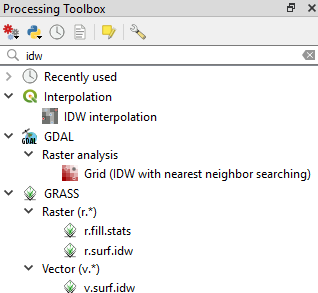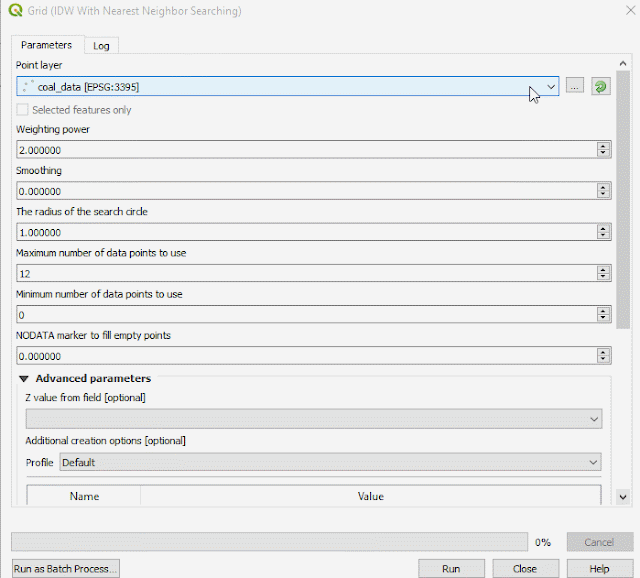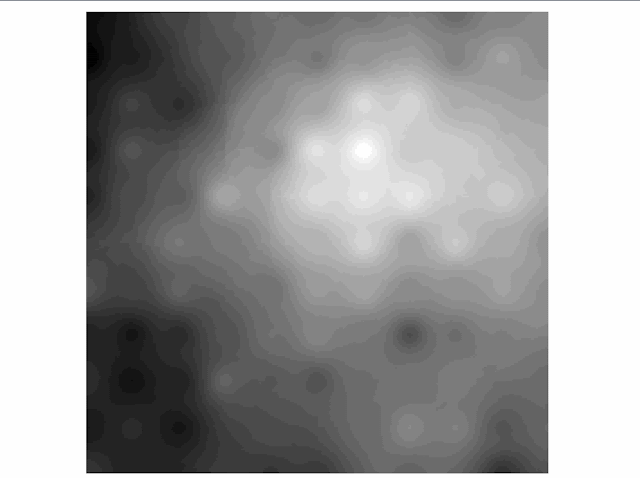# Spatial Interpolation with Inverse Distance Weighting (IDW) Method Explained

As human that is living on the Earth we are always trying to understand the earth itself which is hidden, blur, invisible and complex. Using our knowledge and technology we want to reveal the hidden value or phenomena, so we can take advantage from it. To make things more clear, let's answering a question: Why do we use interpolation? To explain the answer, I will give an example in mineral deposit. Mineral deposit is spatially distributed on the ground. It might be visible in some patterns but mostly invisible below the ground. Then a mining company investigate it to estimate the amount of the mineral deposit and later to define if it is feasible or not to mine. Nobody can give an exact answer how much the deposit, but using a systematic approach and methodology like spatial interpolation at least we can get a close answer from the truth. Next questions: What is spatial interpolation?  How it works? How to use it? I hope this post and tutorial could give the answer and a better explanation.

### Spatial Interpolation Definition and Methods

Interpolation is a method to predict an unknown from known values. From the definition,  we need some known values to do an interpolation using any interpolation method. The known values which is commonly called sampling point, can be gathered from some measurements and site investigation like drilling, surveying, etc. Using the known value from some locations, we are trying to predict a value of other neighborhood location that is close to the known location.

There are many interpolation methods available from a simple to a sophisticated one, some to be named are: linear interpolation, Inverse Distance Weighting (IDW) and Kriging. One method could be differed from each other and could give different results. That's why it is very important to understand how a spatial interpolation works, so we can understand how the result is produced, in what condition to apply it, in what way to apply it to get a better result, what errors could we get, etc. In this post we will discuss a spatial interpolation method which is called Inverse Distance Weighting (IDW). We will see how it works and how to apply it using QGIS 3 software.

### Inverse Distance Weighting(IDW) Interpolation Method

Inverse Distance Weighted interpolation is a deterministic spatial interpolation approach to estimate an unknown value at a location using some known values with  corresponding weighted values. The basic IDW interpolation formula can be seen in equation 1. Where x* is unknown value at a location to be determined, w is the weight,  and x is known point value. The weight is inverse distance of a point to each known point value that is used in the calculation. Simply the weight can be calculated using equation 2.
$x^*=\frac{w_1x_1+w_2x_2+w_3x_3+....+w_nx_n}{w_1+w_2+w_3+...+w_n}$eq 1. Inverse Distance Weight formula
$w_1=\frac{1}{d_{ix^*}^p}$ eq 2. Weight Formula

If we see the formula in equation 2, there is a P variable which stands for Power. There is no particular rule in defining the P value, but from the equation, we can see that the higher P value will give lower weight. From my experience the optimum P value is in range 1 to 2. Of course it can not apply to each case. For more scientific application I suggest to do a little experiment in defining the optimum P value. It could be done by taking a small portion of sample point as testing/validation dataset. Then start with a small P value, do the IDW interpolation and calculate the Root Mean Square Error(RMSE) between the interpolation result and the actual sampling value. Iterate it by increasing the P value step by step and calculate the RMSE. The lowest RMSE is the optimum P value which is given the smallest error between the interpolation and actual value.

Figure 1 gives the illustration how the IDW interpolation works. Can be seen in the figure, a value at position x will be determined from sampling points 1, 2, and 3, with the distances to x point are d1x, d2x and d3x. Using the equation 2, each respective weight will be calculated and then the value at position x will be determined using equation 1.Figure 1. Inverse Distance Weight(IDW) Interpolation

### IDW Interpolation Implementation in GIS Software

Now we are having a basic understanding how the IDW is working. Next question. How this IDW interpolation is implemented in a GIS software? The main problem in implementing the IDW interpolation into a software algorithm is to define how many sampling points will be used in the calculation. This can be done with two approaches, using a number of points and radius distance from a point to be determined (point x). For the first approach, a user can define how many points around x point will be used in the calculation process, so it needs an algorithm to calculate a number of closest point to the x point. The second one, a user can specify a radius distance from point x, then the algorithm must select a number of sampling points within the specified radius.

What about the Power (P) value? The procedure for defining an optimum P value can be done using cross validation method to find the minimum RMSE between interpolation result and actual values as I explained before. If you are using Geostatistics Analyst in ArcGIS software, it will automatically attempt to select an optimal value. Unfortunately not all GIS software provide an automatic algorithm in defining an optimum P value, so you must do it manually.

### How to Perform IDW Interpolation in QGIS

Now I will show you how to do IDW interpolation in QGIS software. For this tutorial I'm using QGIS 3.4.2 Madeira. If you don't have it, you can download QGIS from QGIS official website. The installation of QGIS software is quite simple and straight forward. Read my post about QGIS Introduction that explain more detail about it.

In this tutorial we will use the Coal dataset. It's a simulated dataset based on real coal seam in Southern Africa, which is a companion dataset for Practical Geostatistics text book by Isobel Clark. The dataset contains coal seam thickness in meter, calorific value in Mega Joules/tonne, ash content and sulphur content in %. You can download the Coal data at Kriging coal dataset.

After downloading the coal data, you must do some cleaning using a text editor software like Notepad or Notepad++. Delete two top lines because we don't need it. It just a short information about the data. Then change the column name separator from tab to comma(,). The data must be look like figure 3.Figure 2. Original coal datasetFigure 3. Coal dataset after editing

After the dataset is ready, let's add it to QGIS map canvas. Select Datasource Manager, then select Delimited Dataset. On the Filename, browse and select the coal data. Because the dataset is separated with comma, make sure to select Comma under File Format. Next specify the field for Point coordinates which is column X co-ordinate and Y co-ordinate for X and Y. The coordinates are in meter in local system (I don't find any information which coordinate system is used in the dataset, so I assumed it used local coordinate system). Because there is no information about coordinate system for the dataset, I chose World Mercator Coordinate System (EPSG: 3395).  Below the Sample data preview, you can see how the data looks like. If you see the data are separated well for each column, then everything is fine. Lastly select Add button to add the data into QGIS and close the Datasource Manager window.Figure 4. Add data set into QGIS

#### Export Data to Shapefile Format

After the data is loaded into QGIS. Export the data into shape file format. It can be done by right click the point layer then select  Export >> Save Feature As. The Save Vector Layer as... window will appear. Select the ESRI Shapefile for Format. In the File name specify a name and place where the exported data will be saved. For CRS use the same as previous one, World Mercator.Figure 5. Export coal data to shape file

#### Performing IDW Interpolation in QGIS

Now, we are ready to do the IDW interpolation. In QGIS we can do the IDW interpolation using three tools, there are: IDW Interpolation from QGIS Interpolation tool. v.surf.idw from GRASS and GRID(IDW Nearest Neighbor Searching) from GDAL. If you type a keyword IDW, those three tools will appear in the processing toolbox as in figure 6.Figure 6. IDW available tools in QGIS
Of course you can select one of them, but I prefer to use the IDW tool from GRASS or GDAL, due to more options available like how many sampling points to be used, radius distance, cell size, etc. Compared to default QGIS IDW Interpolation tool which just gives less setting like P value, and number of  output rows and columns.

For this tutorial I'm using IDW interpolation tool from GDAL. From the processing toolbox, open the GRID (IDW with nearest neighbor searching) under GDAL tool as in figure 6.  When you open the tool then the GDAL IDW interpolation window will appear as in figure 7. In the Point layer make sure you select the correct point dataset to be interpolated. Then you can set some parameters like Weighting Power (P value). Smoothing (higher value will give smoother result, default 0). Search radius (I gave it 300) in dataset unit, in this case meter. Don't leave this option 0, cause it won't search any point although you set a number of maximum points. Next, specify the Z value, which is the field value to be interpolated. I want to make a coal calorific map, so I chose the corresponding field. Then at bottom of the window tool, you can specify a place where the output will be saved. If you want to view the result before you save it, leave it as temporary file. Finally click Run button to start the interpolation. When done the result will be added to your QGIS map canvas.Figure 7. GDAL IDW Interpolation Tool
The result in QGIS will be in grayscale mode. To make it more beautiful just customize the symbology. To do it, right click the output result and select Properties (Or simply you can double click the layer name). The Layer Properties window will appear as in figure 8. Select Symbology. In the Band Rendering option change the Render type to Singleband pseudocolor. Right away the output value will be classified into some classes with different color. Of course you can change the number of classes or change the color, just explore if you want to.Figure 8. Change Symbology
Congratulation! You have made a coal calorific map using Inverse Distance Weight (IDW) with QGIS Software.

#### Comparing The IDW Interpolation Result

Before closing thi post, I want to share the result of the IDW interpolation using all the three tools. The three results can be seen in the figure 9.Figure 9. IDW comparison result
Generally, the result from the three IDW interpolation tools produce the same pattern or trend. But visually from the result comparison we can observe that the IDW interpolation using GRASS or GDAL are much more the same to each other compare to IDW interpolation using QGIS interpolation tool. It could happen because I used the same number of interpolation points. For QGIS IDW interpolation it seems high and lower value are clustered closely around the point, so we can see that the higher and lower value concentrate and formed circular area around the points. This could happen because of the stronger influence of the point, due to less distance and number of interpolation point that involved in interpolation processing.

Hopefully this post and tutorial about spatial interpolation using Inverse Distance Weighted (IDW) can give you a better understanding what spatial interpolation is, how it works and how to perform the interpolation using free GIS software (QGIS). As  I mentioned at the beginning of this post, there are some spatial interpolation methods available. Next time I will try to discuss a famous spatial interpolation method Kriging. Thanks for reading and really appreciate your feedback. Anyway there is also a post about how to create IDW algorithm in Python from scratch, check it out if you're interested.